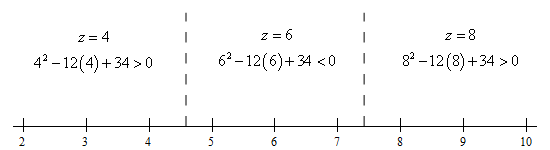Paul's Online Notes
Home / Algebra / Solving Equations and Inequalities / Polynomial Inequalities
Show Mobile Notice Show All Notes Hide All Notes
Mobile Notice
You appear to be on a device with a "narrow" screen width (i.e. you are probably on a mobile phone). Due to the nature of the mathematics on this site it is best views in landscape mode. If your device is not in landscape mode many of the equations will run off the side of your device (should be able to scroll to see them) and some of the menu items will be cut off due to the narrow screen width.

### Section 2-12 : Polynomial Inequalities

4. Solve the following inequality.

${z^2} + 34 > 12z$

Show All Steps Hide All Steps

Start Solution

The first thing we need to do is get a zero on one side of the inequality and then, if possible, factor the polynomial.

${z^2} - 12z + 34 > 0$

In this case the polynomial doesn’t factor.

Hint : Where are the only places where the polynomial might change signs?
Show Step 2

Despite the fact that this is an inequality we first need to know where the polynomial is zero. Because the polynomial didn’t factor we’ll need to use the quadratic formula to determine where it’s zero.

$z = \frac{{12 \pm \sqrt {144 - 4\left( 1 \right)\left( {34} \right)} }}{{2\left( 1 \right)}} = \frac{{12 \pm \sqrt 8 }}{2}\hspace{0.25in} \Rightarrow \hspace{0.25in}z = 4.5858,\,\,7.4142$

We’ll need these points in decimal form to make the rest of the problem easier.

Remember that these points are important because they are the only places where the polynomial on the left side of the inequality might change sign. Given that we want to know where the polynomial is positive knowing where the polynomial might change sign will help considerably with determining the answer we’re looking for.

Hint : Knowing that the polynomial can only change sign at the points above how can we quickly determine if the polynomial is positive or negative in the ranges between those points?
Show Step 3

Recall from the discussion in the notes for this section that because the points from the previous step are the only places where the polynomial might change sign we can quickly determine if the polynomial is positive/negative in the ranges between each of these points simply by plugging in “test points” from each region into the polynomial to check the sign.

So, let’s sketch a quick number line with the points where the polynomial is zero graphed on it. We’ll also show the test point computations on the number line as well. Here is the number line.Show Step 4

All we need to do now is get the solution from the number line in the previous step. Here is both the inequality and interval notation from of the answer.

$\require{bbox} \bbox[2pt,border:1px solid black]{\begin{array}{c}z < 4.5858\hspace{0.25in}{\mbox{and }}\hspace{0.25in}z > 7.4142\\ \left( { - \infty ,4.5858} \right)\hspace{0.25in}{\mbox{and }}\left( {7.4142,\infty } \right)\end{array}}$